# Sum of money

On a certain sum of money, invested at the rate of 10% compounded annually, the difference between the interests of the first year and the third year is 315 . Find the sum.

x =  1363.6364

### Step-by-step explanation:

(1.1^3-1)x -0.1x = 315

(1.1^3-1)•x -0.1•x = 315

0.231x = 315

x = 15000/11 ≈ 1363.636364

Our simple equation calculator calculates it.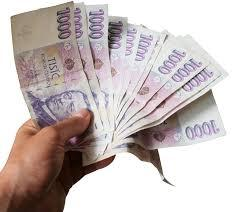Did you find an error or inaccuracy? Feel free to write us. Thank you!Tips to related online calculators
Do you have a linear equation or system of equations and looking for its solution? Or do you have a quadratic equation?

## Related math problems and questions:

• A man 2A man divides \$10,000 into two investments, one at 10% and the other at 30%. Find how much is invested at each rate so that the two investments produce the same income annually.
• Annual interest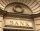A loan of 10 000 euro is to be repaid in annual payments over 10 years. Assuming a fixed 10% annual interest rate compounded annually, calculate: (a) the amount of each annual repayment (b) the total interest paid.
• Apartments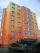Apartment on the first floor was 10% more expensive than the same apartment on the second floor. The difference was 105 Kc annually. Calculate the annual rent of the apartment in the first floor and from apartment on the second floor.
• The crime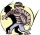The crime rate of a certain city is increasing by exactly 7% each year. If there were 600 crimes in the year 1990 and the crime rate remains constant each year, determine the approximate number of crimes in the year 2025.
• Volume of woodEvery year, at the same time, an increase in the volume of wood in the forest is measured. The increase is regularly p% compared to the previous year. If in 10 years the volume of wood has increased by 10%, what is the number p?
• Machine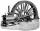Price of the new machine is € 62000. Every year is depreciated 15% of residual value. What will be the value of the machine after 3 years?
• Common ratio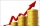If 200 units of a commodity are consumed in a first year, and if the annual rate of increase in consumption is 5% (a) what amount is consumed in the 8th year; (b) in the first 15 years?
• Car downSarah buys a car costing \$12,500. It depreciates value by 8% in the first year, 10% in the second year, and 5% in the third year. Calculate the value of a car after the third year.
• Future valueSuppose you invested \$1000 per quarter over a 15 years period. If money earns an anual rate of 6.5% compounded quarterly, how much would be available at the end of the time period? How much is the interest earn?
• 3y inflation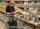Price of the roll rise in the first year by 9%, the second year fell by 5% and in the third year increased by 3%. Calculate the average annual increase in price of the roll.
• Car repair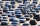John bought a car for a certain sum of money. He spent 10% of the cost to repairs and sold the car for a profit of Rs. 11000. How much did he spend on repairs if he made a profit of 20%?
• RepairingThree employees earned a total of € 469 for repairing the equipment. They split so that the first got 20% more than the second, and the third 15% more than the second. How many euros did everyone get?
• Investment1000\$ is invested at 10% compound interest. What factor is the capital multiplied by each year? How much will be there after n=12 years?
• Suppose 3Suppose that a couple invested Php 50 000 in an account when their child was born, to prepare for the child's college education. If the average interest rate is 4.4% compounded annually, a, Give an exponential model for the situation b, Will the money be
• Retirement annuity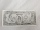How much will it cost to purchase a two-level retirement annuity that will pay \$2000 at the end of every month for the first 10 years, and \$3000 per month for the next 15 years? Assume that the payment represent a rate of return to the person receiving th
• Four numbersThe first number is 50% second, the second number is 40% third, the third number is 20% of the fourth. The sum is 396. What are the numbers?
• DepositOh I total of \$15,000 deposited into simple interest accounts the annual simple interest rate on one account at 6% the annual simple interest rate on the second account at 7% how much should be invested in each account so that the total interest earned is